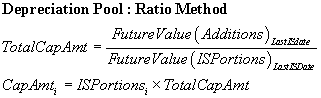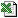DepPoolRatio (Time, Base, FromDatesInt, IntSimple, AdditionDates, Additions, ISDates, ISPortions, LifeOrDecRate, DepType, [FinalValue], [DepSwitch], [DayCount], [Periods], [DayCountDisc], [PrdsDisc])
Operate a Depreciation Pool using the Ratio method
Calculates the ongoing depreciation, written down and WIP value for a depreciation stream using the Ratio Method
Auto Array Function
Mathematical FormulaMethodology

There are three functions in this family that operate a depreciation pool: DepPool, DepPoolRatio and DepPoolWIP.
A depreciation pool is where a series of costs for an asset are progressively accumulated in WIP (work in progress), and then subsequently depreciated on the date(s) when an asset goes into service. The asset cost, which is accumulating in WIP, can accrue interest, and there can be several dates at which a portion of the asset goes into service, each portion having its own depreciation stream. The reason for having several dates when a portion of the asset goes into service is simply to reflect reality, when a producing asset doesn"t go into use on one date, but rather over a period of time as it is fully commissioned.
The 3 functions relate to the 2 methods of operating a depreciation pool in common use. DepPoolWIP uses the relatively simple WIP method, DepPoolRatio uses the more complex but widely used Ratio accounting method; and DepPool uses either method depending on whether you specify any ISPortions or not.

For Both Methods: The costs, or Additions as they are called, are added to the WIP on the AdditionDates. They optionally accrue interest at IntSimple using a daycount of DayCountDisc and a compounding frequency given by PrdsDisc.

For the WIP Method: On each of the in-service dates, or ISDates, whatever is in WIP is crystallised, capitalised and commences its own depreciation stream. The WIP then accumulates, starting at zero, any new Additions, again compounding the interest. At the next ISDate, the WIP is cleared again and depreciated, and so on until the last of the ISDates.

For the Ratio Method: The deficiency with the WIP method is that the amount going into service on each in-service date is a bit arbitrary - it"s whatever is in WIP at the time, rather than relating in some way to the portion of the asset that has really gone into service. The Ratio method defines a set of proportions, or ISPortions, of the ultimate value of the asset, that go into service on each of the ISDates. On each in-service date the relevant portion of the total (ie future, or final value) is withdrawn from WIP and depreciated. Whatever is left in WIP accrues interest, alongside any new Additions. Computationally this method is much harder to do on a spreadsheet since, depending how you do it, it probably creates a degree of circularity - you don"t know how much to depreciate on the first in-service date until of you"ve calculated the total amount to be depreciated, which depended on that first amount that was taken out of WIP and depreciated.

Note: DepPool, the main function, simply calls DepPoolRatio or DepPoolWIP, depending on the existence of ISPortions. DepPool doesn"t do any calculation itself, it just calls one of the two calculation functions.

Tip

DepPool, DepPoolWIP and DepPoolRatio do a lot of internal calculation, and have been greatly optimised for use as Array Functions, which greatly reduces the amount of unnecessary recomputation. We strongly recommend using these three functions as array functions - they are possibly 3 times faster used this way.

Tip

When it comes to computation, this function needs to traverse internally each date in implied by PrdsDisc, for every appropriate year. This is so that any compounding is done accurately with respect to the slight irregularity in time length that occurs from period to period. You can, in many circumstances, make the function go faster, at the cost of a very slight degree of accuracy, by converting the interest rates to annual equivalent rates (AER"s) using SimpleToAER and then using a PrdsDisc of -1.

ExampleDepPoolRatio.xls

Business Functions Ltd, London, UK Website Design: Webpure